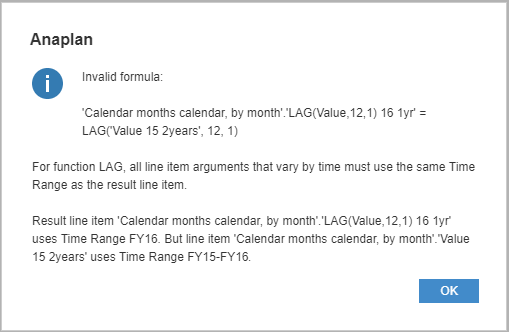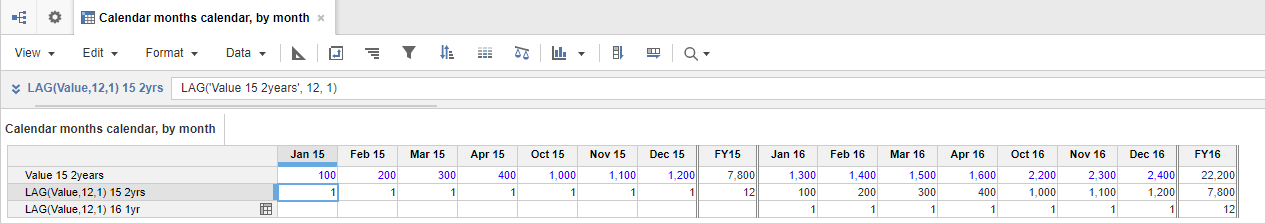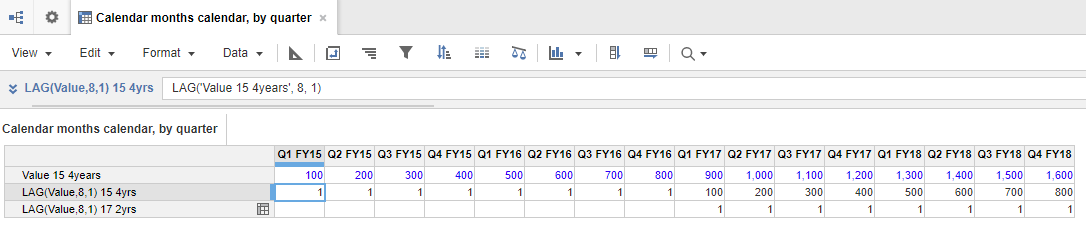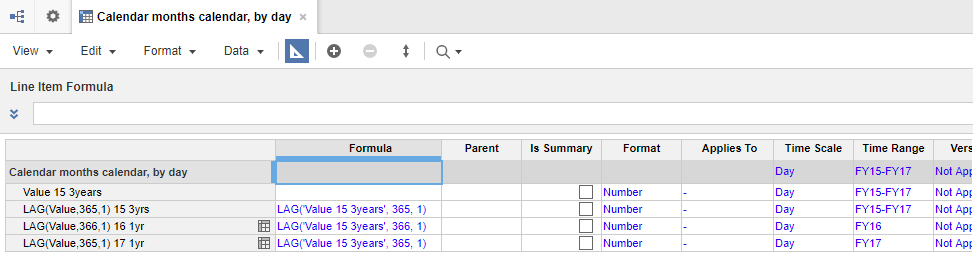1. Modeling
2. Dimensions
3. Time
4. Time Ranges
5. Time Ranges and Calculation Functions
6. Time Ranges and Time Series Functions

Time series functions must use the same time range for the result line item and any line items that provide arguments.

The time series functions are:

• PREVIOUS
• POST
• LAG
• NEXT
• MOVINGSUM
• CUMULATE
• PROFILE
• DECUMULATE
• OFFSET

There is a conformity requirement for all time series functions except Previous and Next. The Invalid formula message displays where the conformity requirement is breached.Anaplan prevents you entering a calculation that uses different time ranges for the line items that provide arguments and the result line item for any of the time series functions.

## Time Series functions in sub-expressions

For time series functions, all referenced line items that vary by time must conform to the result line item (must use the same time range as the result line item). This includes all line items referenced in sub-expressions. Line items referenced by a normal (non-time series) function need not conform, unless the function appears as a sub-expression of a time series function. For example:

1. Result1 = LAG ( Value , Offset , 0 ) + MIN ( Base , Default )
Time ranges of Value and Offset must conform to time range of Result.
Base and Default could use any time range.

2. Result2 = LAG ( Value , MIN ( Offset1 , Offset2 ) , 0 )
Time ranges of Value, Offset1 and Offset2 must conform.

## Hard-coded Offset

Generally for time series functions the time ranges of the result line item and all line item arguments must conform.

There is an exception to this, with the functions LEAD, LAG, OFFSET, and POST. For these functions, the value argument can have a different time range, if:

• Offset argument is a value (a hard-coded number, rather than a reference to a line item).
• Offset value is greater than or equal to the number of periods in result time range.

The system tests whether the offset value points to periods entirely outside the time range of the result item. If this is the case, the value argument can have any time range, and the functions then return the value specified by the fill argument (or zero in the case of POST, which does not have a fill argument).

Note that for LEAD/LAG and OFFSET, the fill argument must match – a calculation can never be applied if the fill and result line items use different time ranges.

### Function syntax

These functions all use an offset argument (n) with a line item (x). The fill value (z) is returned when the offset argument points outside the timescale.

Function Syntax
LAG  LAG(x, n, z,[mode])
OFFSET OFFSET(x,n,z)
POST POST(x, n)
Note: When offset is written in lower case, it refers to the argument that determines the number of periods before or after the current one, not the OFFSET function, which is capitalized.

### Hard-coded offset parameter examples

#### Example 1 - Calendar months calendar, by month

This example has two time ranges.

 Time range Start Year Length FY15-FY16 FY15 2 FY16 FY16 1

The example has three line items.

 Line item Formula Time range Value 15 2years FY15-FY16 LAG(Value,12,1) 15 2yrs LAG(‘Value 15 2Years’,12,1) FY15-FY16 LAG(Value,12,1) 16 1yr LAG(‘Value 15 2Years’,12,1) FY16

The module looks like this:The hard-coded offset (12) in the second line item is equal to the periods in the result time range. This returns values from FY15 to FY16; the Jan 15 value is shown in Jan 16. This is standard behavior.

The one year time range applied to third line item means the hard-coded offset points to periods entirely outside the time range of the result item and causes the function to return the fill value. This is the exceptional behavior.

The time range Invalid formula message would be displayed if the offset argument was a reference to a line item, you would see the dialog:

• when editing a time range (or model calendar).
• if you add a year to the time range used by the third line item LAG(Value,12,1) 16 1yr in the example above.

The dialog displays the invalid formula to help you diagnose the issue. You can then create a valid formula by ensuring line items that provide arguments to the function in the dialog use the same time range as the line item in which the formula is used.

#### Example 2 - Calendar months calendar, by quarter

This example has two time ranges.

 Time range Start Year Length FY15-FY18 with Qtrs FY15 4 FY17-FY18 with Qtrs FY17 2

The example has three line items.

 Line item Formula Time Scale Time range Value 15 4years None Quarter FY15-FY18 with Qtrs LAG(Value,8,1) 15 4yrs LAG(‘Value 15 4Years’,8,1) Quarter FY15-FY18 with Qtrs LAG(Value,8,1) 17 2yrs LAG(‘Value 15 4Years’,8,1) Quarter FY17-FY18 with Qtrs

The module looks like this:The hard-coded offset value, 8, matches the number of quarter periods in result time range.

The third line item returns the fill value.

##### Invalid formula dialog

The Invalid formula dialog is displayed if:

• the hard-coded offset value is between -7 and +7.
• the Time Scale of the third line item is changed to Month, then the hard-coded offset no longer uses periods outside the result line item time range. This can happen if the aggregation is changed directly, or if Quarter Totals are disabled for the time range, which would set line item aggregation to Month.

#### Example 3 - Calendar months calendar, by day

A hard-coded offset value may point to periods outside the result item time range if the actual number of periods varies over the span of the result time range. This can occur when your result refers to financial years.

This example has three time ranges.

 Time range Start Year Length FY15-FY17 FY15 3 FY16 FY16 1 FY17 FY17 1

The example has four line items.

 Line item Formula Time range Value 15 3years FY15-FY17 LAG(Value,365,1) 15 3yrs LAG(‘Value 15 3yrs’,365,1) FY15-FY17 LAG(Value,366,1) 16 1yr LAG(‘Value 15 3yrs’,366,1) FY16 LAG(Value,365,1) 17 1yr LAG(‘Value 15 3yrs’,365,1) FY17

The module looks like this:The second line item does not raise the error because the result and source line items use same time range.

The third line item does not raise the error because hard coded offset is greater than or equal to the number of days in the time range of the result item

The fourth line item returns data from 2015. The hard-coded offset of 365 is accepted because 2015 is not a leap year.

##### Invalid formula message

You’d see the Invalid formula message if you:

• edited time range FY17 to use a leap year.
• changed the offset value in line item LAG(Value, 366, 1) 16 1yr to 365.

#### Example 4 - Weekly calendar types

With weekly calendar types, there is a variable number of weeks (and hence days) in a fiscal year.

A one-year time range could have either 52 or 53 weeks, and either 364 or 371 days. The threshold for a hard-coded offset argument is determined by the actual number of periods in the result time range. So, for example, with weekly granularity and a one-year result time range a hard-coded offset value of 53 or greater would be accepted, whereas a value of 52 may or may not be, depending on the year of the result time range.